# R语言POT超阈值模型在洪水频率分析中的应用研究

### 对于这些同样面临挑战的人，我希望这个博客将有助于简化工作。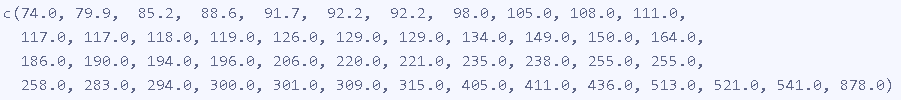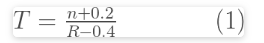• ​T给定排放超标之间的平均间隔（年）
•  R是POT系列中的流量等级（最大流量是等级1）
• ​ n是数据的年数。

R语言极值理论EVT：基于GPD模型的火灾损失分布分析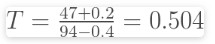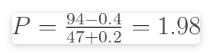ARR示例将指数分布拟合为概率模型。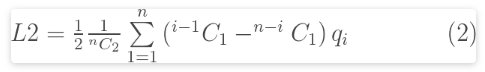``````L2 <- function(q){
q <- sort(q)
n <- length(q)
0.5*(1/choose(n,2))*sum((0:(n-1) - (n-1):0)*q)
}``````

•​ qi从最小到最大的顺序是流量（POT）
•​ n是流的数量

L2 = 79.12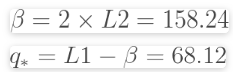Beta的95％置信区间是（37.4，89.4）和​（120.6，244.7）。参数之间的相关性约为-0.5。参数的不确定性如图1所示。

`````` param_errors_df %>%
ggplot(aes(x = V1, y = V2)) +
geom_point(size = 0.1) +
scale_x_continuous(name = 'beta') +
scale_y_continuous(name = bquote('q'['*'])) +
stat_ellipse(colour = 'red') + # 95% 置信区间
theme_gray(base_size = 7)``````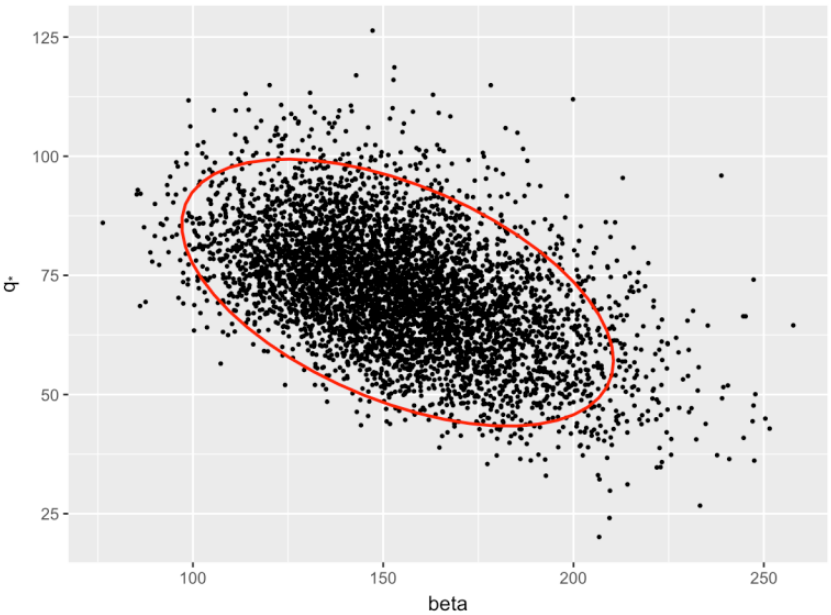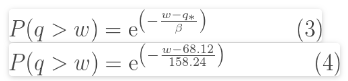74 m 3 / s阈值，POT系列中有47个值，并且有47年的数据，因此每年的平均峰值数为1。因此，EY可以表示为：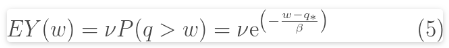w也可以与EY以下内容相关  ：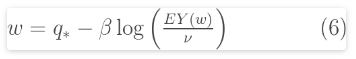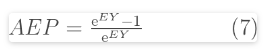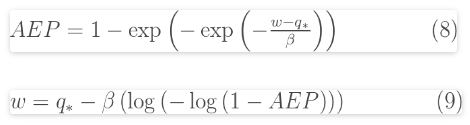``````

res``````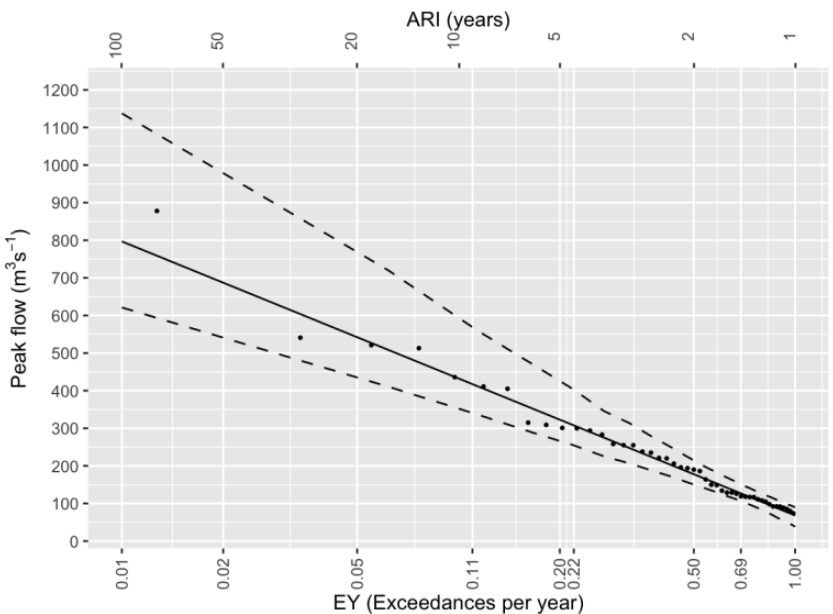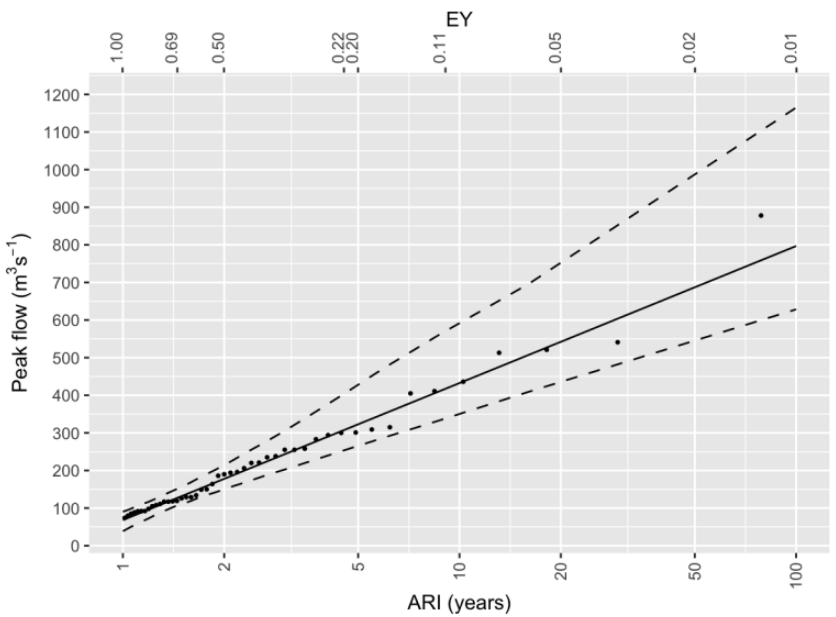Kaizong Ye拓端研究室（TRL）的研究员。

​非常感谢您阅读本文，如需帮助请联系我们！QQ在线咨询

15121130882

0571-63341498

## 关注有关新文章的微信公众号

This will close in 0 seconds